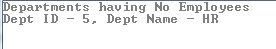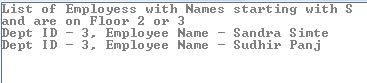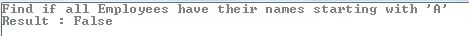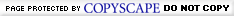# LINQ - Using Quantifiers like Any, Contains and All while Querying Objects

Posted by: Suprotim Agarwal , on 3/2/2009, in Category LINQ
Views: 76809
Abstract: Quantifier operations return a Boolean value if some or all of the elements in a sequence satisfy a condition. In this article, we will see some common LINQ to Objects scenarios where we can use these operators.
LINQ - Using Quantifiers like Any, Contains and All while Querying Objects

Quantifier operations return a Boolean value if some or all of the elements in a sequence satisfy a condition. In this article, we will see some common LINQ to Objects scenarios where we can use these operators.
There are 3 Quantifiers operations that can be used in LINQ:
All – used to determine whether all the elements in a sequence satisfy a condition.
Any - used to determine whether any elements in a sequence satisfy a condition.
Contains - used to determine whether a sequence contains a specified element
Let us see an example that explains these operators and their usages.
I have created a Department and Employee class. Each Employee belongs to a Department. We will query these objects and retrieve information using LINQ through the quantifiers available to us. Here’s the sample data:
C#
static void Main(string[] args)
{
List<Department> dept = new List<Department>();
dept.Add(new Department() { DeptID = 1, DeptName = "Marketing", Floor = 1 });
dept.Add(new Department() { DeptID = 2, DeptName = "Sales", Floor = 2 });
dept.Add(new Department() { DeptID = 3, DeptName = "Administration", Floor = 3 });
dept.Add(new Department() { DeptID = 4, DeptName = "Accounts", Floor = 3 });
dept.Add(new Department() { DeptID = 5, DeptName = "HR", Floor = 3 });

List<Employee> emp = new List<Employee>();
emp.Add(new Employee() { EmpID = 1, DeptID = 1, EmpName = "Jack Nolas"});
emp.Add(new Employee() { EmpID = 2, DeptID = 4, EmpName = "Mark Pine" });
emp.Add(new Employee() { EmpID = 3, DeptID = 3, EmpName = "Sandra Simte" });
emp.Add(new Employee() { EmpID = 4, DeptID = 4, EmpName = "Larry Lo"});
emp.Add(new Employee() { EmpID = 5, DeptID = 3, EmpName = "Sudhir Panj" });
emp.Add(new Employee() { EmpID = 6, DeptID = 2, EmpName = "Kathy K" });
emp.Add(new Employee() { EmpID = 7, DeptID = 1, EmpName = "Kaff Joe" });
emp.Add(new Employee() { EmpID = 8, DeptID = 1, EmpName = "Su Lie" });
}
class Department
{
public int DeptID { get; set; }
public string DeptName { get; set; }
public int Floor { get; set; }
}

class Employee
{
public int EmpID { get; set; }
public int DeptID { get; set; }
public string EmpName { get; set; }
}
VB.NET
Sub Main(ByVal args() As String)
Dim dept As New List(Of Department)()
dept.Add(New Department() With {.DeptID = 1, .DeptName = "Marketing", .Floor = 1})
dept.Add(New Department() With {.DeptID = 2, .DeptName = "Sales", .Floor = 2})
dept.Add(New Department() With {.DeptID = 3, .DeptName = "Administration", .Floor = 3})
dept.Add(New Department() With {.DeptID = 4, .DeptName = "Accounts", .Floor = 3})
dept.Add(New Department() With {.DeptID = 5, .DeptName = "HR", .Floor = 3})

Dim emp As New List(Of Employee)()
emp.Add(New Employee() With {.EmpID = 1, .DeptID = 1, .EmpName = "Jack Nolas"})
emp.Add(New Employee() With {.EmpID = 2, .DeptID = 4, .EmpName = "Mark Pine"})
emp.Add(New Employee() With {.EmpID = 3, .DeptID = 3, .EmpName = "Sandra Simte"})
emp.Add(New Employee() With {.EmpID = 4, .DeptID = 4, .EmpName = "Larry Lo"})
emp.Add(New Employee() With {.EmpID = 5, .DeptID = 3, .EmpName = "Sudhir Panj"})
emp.Add(New Employee() With {.EmpID = 6, .DeptID = 2, .EmpName = "Kathy K"})
emp.Add(New Employee() With {.EmpID = 7, .DeptID = 1, .EmpName = "Kaff Joe"})
emp.Add(New Employee() With {.EmpID = 8, .DeptID = 1, .EmpName = "Su Lie"})
End Sub

Friend Class Department
Private privateDeptID As Integer
Public Property DeptID() As Integer
Get
Return privateDeptID
End Get
Set(ByVal value As Integer)
privateDeptID = value
End Set
End Property
Private privateDeptName As String
Public Property DeptName() As String
Get
Return privateDeptName
End Get
Set(ByVal value As String)
privateDeptName = value
End Set
End Property
Private privateFloor As Integer
Public Property Floor() As Integer
Get
Return privateFloor
End Get
Set(ByVal value As Integer)
privateFloor = value
End Set
End Property
End Class

Friend Class Employee
Private privateEmpID As Integer
Public Property EmpID() As Integer
Get
Return privateEmpID
End Get
Set(ByVal value As Integer)
privateEmpID = value
End Set
End Property
Private privateDeptID As Integer
Public Property DeptID() As Integer
Get
Return privateDeptID
End Get
Set(ByVal value As Integer)
privateDeptID = value
End Set
End Property
Private privateEmpName As String
Public Property EmpName() As String
Get
Return privateEmpName
End Get
Set(ByVal value As String)
privateEmpName = value
End Set
End Property
End Class

Using ‘Any’ Quantifier in LINQ
This sample uses the ‘Any’ operator to list down the Departments that do not have Employees
C#
var noEmp =
from d in dept
where !emp.Any(e => e.DeptID == d.DeptID)
select new { dId = d.DeptID, dNm = d.DeptName };

Console.WriteLine("Departments having no Employees");
foreach (var empl in noEmp)
{
Console.WriteLine("Dept ID - " + empl.dId + ", Dept Name - " + empl.dNm);

}
VB.NET
Dim noEmp = _
From d In dept _
Where (Not emp.Any(Function(e) e.DeptID = d.DeptID)) _
Select New With {Key .dId = d.DeptID, Key .dNm = d.DeptName}

Console.WriteLine("Departments having no Employees")
For Each empl In noEmp
Console.WriteLine("Dept ID - " & empl.dId & ", Dept Name - " & empl.dNm)
Next empl
Output:Using ‘Contains’ Quantifier in LINQ
The following example uses the ‘Contains’ quantifier to find the List of Departments having Employee Names starting with ‘S’
C#
// Functionality Similar to IN operator
var hasEmp = dept
.Where(e => emp.Where(contact =>
contact.EmpName.StartsWith("S"))
.Select(d => d.DeptID)
.Contains(e.DeptID));

Console.WriteLine("/nList of Departments having Employee Names starting with S");
foreach (var dpt in hasEmp)
{
Console.WriteLine("Dept ID - " + dpt.DeptID + ", Dept Name - " + dpt.DeptName);
}

VB.NET
' Functionality Similar to IN operator
Dim hasEmp = dept.Where(Function(e) emp.Where(Function(contact) contact.EmpName.StartsWith("S")).Select(Function(d) d.DeptID).Contains(e.DeptID))

Console.WriteLine("List of Departments having Employee Names starting with S")
For Each dpt In hasEmp
Console.WriteLine("Dept ID - " & dpt.DeptID & ", Dept Name - " & dpt.DeptName)
Next dpt

Output:
If you want to add another condition to the above query where only Departments in Floor 2 and 3 are to be considered, then here’s how to do so:
C#
List<int> floorNo = new List<int>() {2, 3};
var floo = emp.Where(contact =>
contact.EmpName.StartsWith("S"))
.Where(du => dept.Where(dp => floorNo.Contains(dp.Floor))
.Select(dp => dp.DeptID)
.Contains(du.DeptID));

Console.WriteLine("List of Employess with Names starting with S\nand are on Floor 2 or 3");
foreach (var dpt in floo)
{
Console.WriteLine("Dept ID - " + dpt.DeptID + ", Employee Name - " + dpt.EmpName);
}

VB.NET
Dim floorNo As New List(Of Integer)(New Integer() {2, 3})
Dim floo = emp.Where(Function(contact) contact.EmpName.StartsWith("S")).Where(Function(du) dept.Where(Function(dp) floorNo.Contains(dp.Floor)).Select(Function(dp) dp.DeptID).Contains(du.DeptID))

Console.WriteLine("List of Employess with Names starting with S" & Constants.vbLf & "and are on Floor 2 or 3")
For Each dpt In floo
Console.WriteLine("Dept ID - " & dpt.DeptID & ", Employee Name - " & dpt.EmpName)
Next dpt

Output:Using ‘All’ Quantifier in LINQ
Using the ‘All’ operator, we can determine whether all employees have their names starting with ‘A’
C#
Console.WriteLine("Find if all Employees have their names starting with 'A'");
bool chkName = emp.All(e =>
e.EmpName.StartsWith("A"));
Console.WriteLine("Result : " + chkName);

VB.NET
Console.WriteLine("Find if all Employees have their names starting with 'A'")
Dim chkName As Boolean = emp.All(Function(e) e.EmpName.StartsWith("A"))
Console.WriteLine("Result : " & chkName)

OutputThose were some common operations using Quantifiers in LINQ. I hope this article was useful and I thank you for viewing it.

C# and .NET have been around for a very long time, but their constant growth means there’s always more to learn.

We at DotNetCurry are very excited to announce The Absolutely Awesome Book on C# and .NET. This is a 500 pages concise technical eBook available in PDF, ePub (iPad), and Mobi (Kindle).

Organized around concepts, this Book aims to provide a concise, yet solid foundation in C# and .NET, covering C# 6.0, C# 7.0 and .NET Core, with chapters on the latest .NET Core 3.0, .NET Standard and C# 8.0 (final release) too. Use these concepts to deepen your existing knowledge of C# and .NET, to have a solid grasp of the latest in C# and .NET OR to crack your next .NET Interview.

AuthorSuprotim Agarwal, MCSD, MCAD, MCDBA, MCSE, is the founder of DotNetCurry, DNC Magazine for Developers, SQLServerCurry and DevCurry. He has also authored a couple of books 51 Recipes using jQuery with ASP.NET Controls and The Absolutely Awesome jQuery CookBook.

Suprotim has received the prestigious Microsoft MVP award for Fifteen consecutive years. In a professional capacity, he is the CEO of A2Z Knowledge Visuals Pvt Ltd, a digital group that offers Digital Marketing and Branding services to businesses, both in a start-up and enterprise environment.Feedback - Leave us some adulation, criticism and everything in between!
 Comment posted by Thanigainathan on Tuesday, July 28, 2009 3:07 AM
Hi,

Article is very nice. But please specify that you have used objects emp and dept in the linq queries.
Becuase its little difficult to udnerstans.

Thanks,
Thani
 Comment posted by uzivatel on Friday, July 31, 2009 8:13 AM
from d in dept
where !emp.Any(e => e.DeptID == d.DeptID)

wouldn't it be better:
where emp.Any(e => e.DeptID != d.DeptID)

thanks
 Comment posted by Ehsan on Friday, July 31, 2009 1:57 PM
Thanks for the article! I would also agree with uzivatel regarding the "!=".  It would be easier to understand.
 Comment posted by Suprotim Agarwal on Wednesday, August 5, 2009 3:55 AM
Uzivatel, Ehsan: Thanks. This will be simpler.
 Comment posted by David Jacob Jarquin on Friday, January 8, 2010 10:46 AM
Contains can also be use in sql subqueries
//FIRST A LIST
//CONTAINS IN A LIST
var query = from c in db.due_dates
select c;